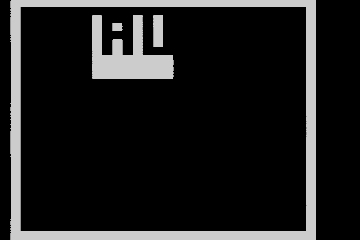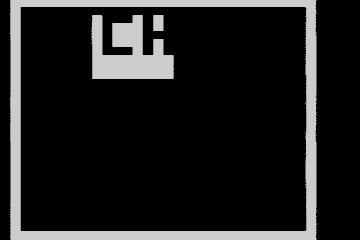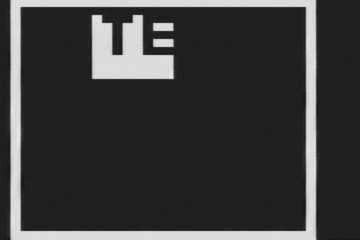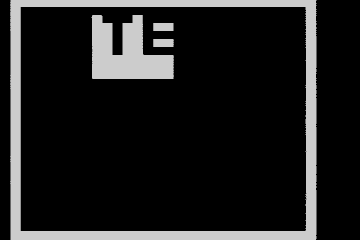# Gzalo

ES · EN

## Mini game console with TV out (2012)

In 2012, a few years after designing and building the Mini game console with LED matrix, I found a very interesting article showing an easy way to generate black and white NTSC signals using cheap microcontrollers. The article itself can be found here.

Using the idea of two resistors to generate 4 values and thus create the sync signal and 3 colors (black, white, gray), I adapted the mini console to have a composite video out. Hardware-wise, it was needed to add a buffer, since the output current of the used microcontroller (AT89S52) is not high enough to drive the signal, which has a 75-ohm impedance at the TV end.

Regarding the software, the fact that multiple televisions and capture cards don’t require the full vertical sync signal was used. In this time, the game logic was executed. Bit accessible registers were used, in order to extract the bits of the frame buffer in an efficient way. For faster output, the UART of the microcontroller may be used in an synchronic mode, and thus improve the horizontal resolution by a factor of ~8. In this prototype, a 30x32 resolution was perfectly achieved. To have a higher resolution, external RAM would be needed.

The working code can be found inside of the same repository that contains the mini game console.

The code used is similar to this one:

``````#define BitSync P1_0
#define BitColor P3_0

__idata __at(0x80) unsigned char frameBuffer[30*4];  //Framebuffer containing the pixels that will be shown
unsigned char line=0,i,j,k;

__bit bit00, bit01, bit02, bit03, bit04, bit05, bit06, bit07,
bit08, bit09, bit10, bit11, bit12, bit13, bit14, bit15,
bit16, bit17, bit18, bit19, bit20, bit21, bit22, bit23,
bit24, bit25, bit26, bit27, bit28, bit29, bit30, bit31;
unsigned char __at(0x20) currentByte;
//The idea is that this 4 bytes should overlap with the bit access segment, so the 32 bits are easily accessable

void hsync(void) __interrupt(TF0_VECTOR) __naked{
__asm
setb	_P1_0
inc	_line
mov	a,_line
rrc	a
anl a,#0x7C		;Vertical offset to improve margin
mov	r1,a
clr	_P1_0

mov	_currentByte,@r1
inc r1
mov	_currentByte+1,@r1
inc r1
mov	_currentByte+2,@r1
inc r1
mov	_currentByte+3,@r1
nop
__endasm;

//We output the current line, in the correct order
BitColor = bit07;
BitColor = bit06;
BitColor = bit05;
BitColor = bit04;
BitColor = bit03;
BitColor = bit02;
BitColor = bit01;
BitColor = bit00;
BitColor = bit15;
BitColor = bit14;
BitColor = bit13;
BitColor = bit12;
BitColor = bit11;
BitColor = bit10;
BitColor = bit09;
BitColor = bit08;
BitColor = bit23;
BitColor = bit22;
BitColor = bit21;
BitColor = bit20;
BitColor = bit19;
BitColor = bit18;
BitColor = bit17;
BitColor = bit16;
BitColor = bit31;
BitColor = bit30;
BitColor = bit29;
BitColor = bit28;
BitColor = bit27;
BitColor = bit26;
__asm;
nop
nop
__endasm;
BitColor = 1;

if(line == 0){
BitSync = 1;
P1_1 = 1;

TR1 = 0;
TH1 = 0xFF-10;
TL1 = 0xF0;
TR1 = 1;
//Using timer1 to count the VSYNC time

gameLogic();	//Run the game logic (assuming that it takes less than VSYNC time)
while(!TF1);	//Wait until VSYNC time is over
TR1 = 0;
TF1 = 0;

BitSync = 0;
P1_1 = 0;
}
__asm;
reti
__endasm;
}

int main(){
unsigned char i;
frameBuffer = frameBuffer = frameBuffer = frameBuffer = 0x00;
frameBuffer = frameBuffer = frameBuffer = frameBuffer = 0x00;
for(i=4;i<116;i+=4)
frameBuffer[i] = 0x7F,frameBuffer[i+1] = 0xFF,
frameBuffer[i+2] = 0xFF, frameBuffer[i+3] = 0xF8;	//Draw border

TMOD = T0_M1 | T1_M0;
TH0 = 128; //Timer0 interrupt every 64 uS (HSYNC)
IT0 = 1;

TR0 = 1;

EA = 1;
ET0 = 1;

SCON = 0;

while(1);
}
``````

Some results may be seen in these animated GIFs, captured using an Aimslab VHX card. The game code is the same used in the small game console, so the game resolution is still 8x8 (it should be adaptable and all the 30x32 pixels can be used)